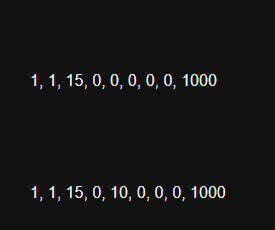# NaN values after adding numbers to initialised array

Twine Version: 2.3.16
SugarCube 2.36.1

Hello
I am creating game in Twine and am using mostly twine assets - I’m not using Java scripts or something like that. If it can be done in twine code, I’m trying to do this.

My problem is, that I use many arrays.
I have initalised several arrays like that:

``````<<set \$mcArkana = []>>
<<set \$mcArkana = 1>>
<<set \$mcArkana = 1>>
<<set \$mcArkana = 15>>
<<set \$mcArkana = 0>>
<<set \$mcArkana = 0>>
<<set \$mcArkana = 0>>
<<set \$mcArkana = 0>>
<<set \$mcArkana = 0>>
<<set \$mcArkana = \$mcArkana * 1000>>
``````

Before that array was lake that (it did’n work either):

``````<<set \$mcArkana = []>>
<<set \$mcArkana = 1>>
<<set \$mcArkana = 1>>
<<set \$mcArkana = 15>>
<<set \$mcArkana = 0>>
<<set \$mcArkana = []>>
<<set \$mcArkana = [0,0,0]>>
<<set \$mcArkana = 0>>
<<set \$mcArkana = \$mcArkana * 1000>>
``````

I created “debug display” of that arrays in StoryCaption, so I have something like that:

`1, 1, 15, 0, 0, 0, 0, 0, 1000`

In 4th, 5th & 6th value (or in array under 4th value before) are date values (minutes, hours and days) I would like to change. So I add value:

`<<set \$mcArkana += 10>> //adding 10 minutes to Arkana timer`

or even:

` <<set \$mcArkana = 10>>`

Right after the addition debug displays:

` 1, 1, 15, 0, [number NaN], [number NaN], [number NaN], 0, 1000`

I know, that this three variables were connected. I dropped this connection, but…

Tried everything.

Kthaara

Hello!

I quickly tested the following:

``````<<set \$mcArkana = []>>
<<set \$mcArkana = 1>>
<<set \$mcArkana = 1>>
<<set \$mcArkana = 15>>
<<set \$mcArkana = 0>>
<<set \$mcArkana = 0>>
<<set \$mcArkana = 0>>
<<set \$mcArkana = 0>>
<<set \$mcArkana = 0>>
<<set \$mcArkana = \$mcArkana * 1000>>

\$mcArkana

<<set \$mcArkana += 10>>

\$mcArkana
``````

And got the following result:Which means that the setting of those variable does work.

Is there maybe another code that affect this variable in your project? maybe an `<<unset>>` macro somewhere?

(If you are creating some code for keeping Time, may I suggest @Hituro 's Date System instead?)

You are setting some values to arrays, not numbers.

An array plus a number will give you NaN (not a number).

Ideally you should either be using the `<array>.push()` function to adding additional elements to an existing Array…

``````<<set \$mcArkana = []>>
<<run \$mcArkana.push(1)>>
<<run \$mcArkana.push(1)>>
<<run \$mcArkana.push(15)>>
<<run \$mcArkana.push(0)>>
<<run \$mcArkana.push(0)>>
<<run \$mcArkana.push(0)>>
<<run \$mcArkana.push(0)>>
<<run \$mcArkana.push(0)>>
<<run \$mcArkana.push(\$mcArkana * 1000)>>
``````

note: I used the `<<run>>` macro instead of `<<set>>` because I find it reads better when executing a JavaScript function, but as `<<run>>` is just an alias for `<<set>>` the two macros are interchangeable.

Or in the case of an Array with a set length, you should be using the `Array(length)` constructor to create an Array with the required number of element…

``````<<set \$mcArkana = Array(9)>>
<<set \$mcArkana = 1>>
<<set \$mcArkana = 1>>
<<set \$mcArkana = 15>>
<<set \$mcArkana = 0>>
<<set \$mcArkana = 0>>
<<set \$mcArkana = 0>>
<<set \$mcArkana = 0>>
<<set \$mcArkana = 0>>
<<set \$mcArkana = \$mcArkana * 1000>>
``````

Hey
It was not related to the array itself but to one tiny function. In this function was a bug, crushed witch mighty `<<if>>` statement.